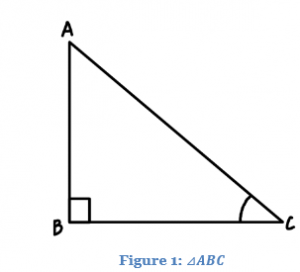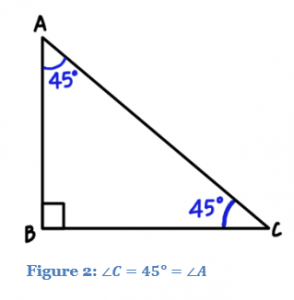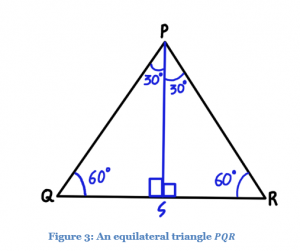# Trigonometric Ratios Of Standard Angles

Trigonometric ratios are Sine, Cosine, Tangent, Cotangent, Secant and Cosecant. The standard angles for these trigonometric ratios are 0°, 30°, 45°, 60° and 90°. These angles can also be represented in the form of radians such as 0, π/6, π/4, π/3, and π/2. These angles are most commonly and frequently used in trigonometry. Learning the values of these trigonometry angles is very necessary to solve various problems.

Trigonometric Ratios Formulas:

The six trigonometric ratios are basically expressed in terms of the right-angled triangle.∆ABC is a right-angled triangle, right-angled at (shown in figure 1). The six trigonometric ratios for ∠C are defined as:

$$sin~∠C$$ = $$\frac{AB}{AC}$$

$$cosec~∠C$$ = $$\frac{1}{sin~∠C}$$

$$cos~∠C$$ = $$\frac{BC}{AC}$$

$$sec~∠C$$ = $$\frac{1}{cos~∠C}$$

$$tan~∠C$$ = $$\frac{sin~∠C}{cos~∠C}$$$$~~~~~~~~~~~~~~~~~~~~~~~~~~~~~~~~$$

$$cot~∠C$$ = $$\frac{1}{tan~∠C}$$

The standard angles for which trigonometric ratios can be easily determined are $$0°,30°,45°,60°$$ and $$90°$$. The values are determined using properties of triangles. The two acute angles of a right-angled triangle are complementary.

## Trigonometric Ratios Table (Standard Angles)

 Angle = ∠C 0° 30° 45° 60° 90° $$sin~C$$ 0 $$\frac{1}{2}$$ $$\frac{1}{\sqrt{2}}$$ $$\frac{\sqrt{3}}{2}$$ 1 $$cos~C$$ 1 $$\frac{\sqrt{3}}{2}$$ $$\frac{1}{\sqrt{2}}$$ $$\frac{1}{2}$$ 0 $$tan~C$$ 0 $$\frac{1}{\sqrt{3}}$$ 1 $$\sqrt{3}$$ Not Defined $$cosec~C$$ Not Defined 2 $$\sqrt{2}$$ $$\frac{2}{\sqrt{3}}$$ 1 $$sec~C$$ 1 $$\frac{2}{\sqrt{3}}$$ $$\sqrt{2}$$ 2 Not Defined $$cot~C$$ Not Defined $$\sqrt{3}$$ 1 $$\frac{1}{\sqrt{3}}$$ 0

The above table shows the important angles for all the six trigonometric ratios. Let us learn here how to derive these values.

## Derivation of Trigonometric Ratios for Standard Angles

Value of Trigonometric Ratios for Angle equal to 45 degreesIn $$⊿ABC$$, if $$∠C$$ = $$45°$$, then $$∠A$$ = $$45°$$. Since the angles are equal, $$⊿ABC$$ becomes a right angled isosceles triangle. So, $$AB$$ = $$BC$$. Assume $$AB$$ = $$BC$$ = $$a$$ units.

Using Pythagoras theorem ,

$$AC^2$$ = $$AB^2~+~BC^2$$

$$AC^2$$ = $$a^2~+~a^2$$

$$AC$$ = $$a\sqrt{2} ~units$$

$$∠C$$ = $$45°$$

$$∴~ sin~∠C$$ = $$sin~45°$$ = $$\frac{AB}{AC}$$ = $$\frac{a}{a\sqrt{2}}$$ = $$\frac{1}{\sqrt{2}}$$ $$~~~~~~~~~~~~~~~~~~~~~~~~~~~~~~~~$$

$$cosec~45°$$ = $$\frac{1}{sin~45°}$$ = $$\sqrt{2}$$

$$cos~∠C$$ = $$cos~45°$$ = $$\frac{BC}{AC}$$ = $$\frac{a}{a\sqrt{2}}$$ = $$\frac{1}{\sqrt{2}}$$   $$~~~~~~~~~~~~~~~~~~~~~~~~~~~~~~~~~~~~~~$$

$$sec~45°$$ = $$\frac{1}{cos~45°}$$ = $$\sqrt{2}$$

$$tan~45°$$ = $$\frac{sin~45°}{cos~45°}$$ = $$\frac{\frac{a}{\sqrt{2}}}{\frac{a}{\sqrt{2}}}$$ = $$1$$$$~~~~~~~~~~~~~~~~~~~~~~~~~~~~~~~~~~~~~~~~~~~~~~~~~~~~~~~~~~~$$

$$cot~45°$$ = $$\frac{1}{tan~45°}$$ = $$1$$

Value of Trigonometric Ratios for Angle equal to 30 and 60 degreesIn figure 3, $$∆PQR$$ is equilateral. The perpendicular from any vertex on the opposite side is coincident with the angle bisector of that particular vertex. Also, the perpendicular bisects the opposite side. If a perpendicular $$PS$$ is dropped on $$QR$$, then $$∠QPS$$ = $$∠SPR$$ = $$30°$$ and $$QS$$ = $$SR$$. Assume $$PQ$$ = $$QR$$ = $$RP$$ = $$2a$$ units.

$$⇒~QS$$ = $$SR$$ = $$a$$ units

In $$∆PSQ$$, by Pythagoras theorem,

$$PQ^2$$ = $$QS^2~+~PS^2$$

$$PS^2$$ = $$(2a)^2~-~a^2$$

$$PS$$ = $$\sqrt{3a^2}$$ = $$\sqrt{3} a$$

$$∠SPQ$$ = $$30°$$

$$sin~∠SPQ$$ = $$sin~30°$$ = $$\frac{SQ}{PQ}$$ = $$\frac{a}{2a}$$ = $$\frac{1}{2}$$   $$~~~~~~~~~~~~~~~~~~~~~~~~~~~~~~~~~~~~~$$

$$cosec~30°$$ = $$\frac{1}{sin~30°}$$ = $$2$$

$$cos~∠SPQ$$ = $$cos~30°$$ = $$\frac{PS}{PQ}$$ = $$\frac{\sqrt{3} a}{2a}$$ = $$\frac{\sqrt{3}}{2}$$    $$~~~~~~~~~~~~~~~~~~~~~~~~~~~~~~~~$$

$$sec~30°$$ = $$\frac{1}{cos~30°}$$ = $$\frac{2}{\sqrt{3}}$$

$$tan~30°$$ = $$\frac{sin~30°}{cos~30°}$$ = $$\frac{\frac{1}{2}}{\frac{\sqrt{3}}{2}}$$ = $$\frac{1}{\sqrt{3}}$$    $$~~~~~~~~~~~~~~~~~~~~~~~~~~~~~~~~~~~~~~~~~~~~~~~~~~~~$$

$$cot~30°$$ = $$\frac{BC}{AB}$$ = $$\sqrt{3}$$

Similarly, ratios of 60° are determined by finding the ratios of $$∠SQP$$ as

$$sin~60°$$ = $$\frac{\sqrt{3}}{2}$$      $$~~~~~~~~~~~~~~~~~~~~~~~~~~~~~~~~~~~~~~~~~~~~~~~~~~~~$$

$$cos~60°$$ = $$\frac{1}{2}$$

$$tan~60°$$ = $$\sqrt{3}$$       $$~~~~~~~~~~~~~~~~~~~~~~~~~~~~~~~~~~~~~~~~~~~~~~~~~~$$

$$cot~60°$$ = $$\frac{1}{\sqrt{3}}$$

$$cosec~60°$$ = $$\frac{2}{\sqrt{3}}$$      $$~~~~~~~~~~~~~~~~~~~~~~~~~~~~~~~~~~~~~~~~~~~~~~~~~~~~$$

$$sec~60°$$ = $$2$$

Value of Trigonometric Ratios for Angle equal to 0 and 90 degrees

In $$∆ABC$$ is a right angled triangle. If the length of side $$BC$$ is continuously decreased, then value of $$∠A$$ will keep on decreasing. Similarly, value of $$∠C$$ is increasing as length of $$BC$$ is decreasing. When BC = 0, ∠A = 0 , ∠C = 90° and AB = AC.

Taking ratios for $$∠A$$ = $$0°$$

$$sin~∠A$$ = $$sin~0°$$ = $$\frac{BC}{AC}$$ = $$\frac{0}{AC}$$ = $$0$$

$$cosec~0°$$ = $$\frac{1}{sin~0°}$$ = $$\frac{1}{0}$$ = Not Defined

$$cos~∠A$$ = $$cos~0°$$ = $$\frac{AB}{AC}$$ = $$\frac{AC}{AC}$$ = $$1$$

$$sec~0°$$ = $$\frac{1}{cos~0°}$$ = $$\frac{1}{1}$$ = $$1$$

$$tan~0°$$ = $$\frac{sin~0°}{cos~0°}$$ = $$\frac{0}{1}$$ = $$0$$

$$cot~0°$$ = $$\frac{1}{tan~0°}$$ = $$\frac{1}{0}$$ = Not Defined

Taking ratios for $$∠C$$ = $$90°$$

$$sin~∠C$$ = $$sin~90°$$ = $$\frac{AB}{AC}$$ = $$\frac{AC}{AC}$$ = $$1$$

$$cosec 90°$$ = $$\frac{1}{sin~90°}$$ = $$\frac{1}{1}$$ = $$1$$

$$cos~∠C$$ = $$cos~90°$$ = $$\frac{BC}{AC}$$ = $$\frac{0}{AC}$$ = $$0$$

$$sec~90°$$ = $$\frac{1}{cos~90°}$$ = $$\frac{1}{0}$$ = Not Defined

$$tan~90°$$ = $$\frac{sin~90°}{cos~90°}$$ = $$\frac{1}{0}$$ = Not Defined

$$cot~90°$$ = $$\frac{1}{tan~90°}$$ = $$\frac{0}{1}$$ = $$0$$

Following is the trigonometric ratios table which contains all the trigonometric ratios of standard angles:

### Solved Examples

Question 1: What is the value of tan 30+sin 60?

Solution: tan 30 = 1/√3 and sin 60 = √3/2

Adding both the values we get;

1/√3  + √3/2

Rationalising the denominator gives:

(2+√3.√3)/2√3

2+3/2√3

5/2√3

Question 2: What is the value of sin45 – cos 45?

Solution: Sin 45 = 1/√2 and cos 45 = 1/√2

Therefore, on putting the values we get:

1/√2 – 1/√2 = 0

## Video Lesson

### Trigonometric Ratios of Compound AnglesTo learn about other topics, visit www.byjus.com. Download BYJU’S – The Learning App to enjoy interactive learning.

Quiz on Trigonometric Ratios of Standard Angles